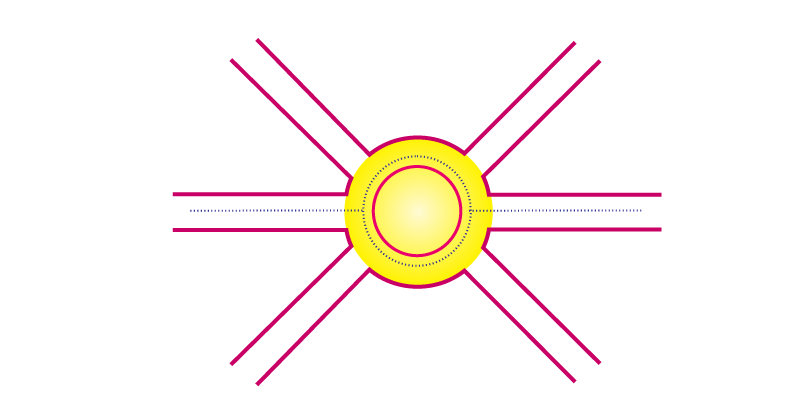# A boy is moving on a straight road against a frictional force of 5 N. After travelling a distance of 1.5 km he forgot the correct path at a round about (Fig.) of radius 100 m. However, he moves on the circular path for one and half cycle and then he moves forward upto 2.0 km. Calculate the work done by him.Total distance travelled by the boy = 1500 m + 200 m + (1.5 x 2 πr)

Total distance travelled by the boy = 1500 m + 200 m + (1.5 x 2 x 3.14 x 100)

Total distance travelled by the boy = 4442 m

Frictional force = 5 N

Workdone = Force × Displacement

W = F × D

Workdone (W) = 5 × 4442

W = 22210 J(0)(0)